Drive_Index

Calculating shaft Deflections

Introduction

This webpage includes notes on methods of calculating radial deflections on shafts transmitting torques and subject to lateral loads and moments which tend to bend the shaft. The notes are provided to enable basic outline calculations to be carried out.    For detailed design work it is recommended that quality reference documents are used.

Online calculators are provided which can also provide convenient methods of evaluating the deflections.     One I have used is Mitcalc. com ( see link below).

Various methods are available for calculating the deflections of a shaft . Many of these are described on pages indexed at webpage Beam index.     The calculation of beam deflections can be simplified by using the principle of superposition .    The deflections resulting from seperate loads in the same plane , or different planes, can be seperately calculated and then combined arithmetically or vectorial to arrive at the deflections of the combined loading scenario.

It should be noted that, in general, there is only a need to determine the radial/angular deflection at the location of a bearing , gear, spline, coupling etc. It is not , generally, necessary to determine the deflection in the mid point of a shaft, in space, assuming that the deflection is not excessive.

A first step is always to determine the forces and moments resulting from gears, belts, weight of components etc and calculate the bearing reactions.    It is generally simpler to calculate these with reference to the horizontal and the vertical planes eg. a force at an angle to the vertical has a component in the horizontal plane and one in the vertical plane.    The reactions are generally calculated using the principles of static equilibrium.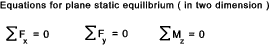Examples of using this principle are found on web page Shear Bending moment diagrams.

Shafts of same diameter

It is often sufficient to calculate the deflections based on the shaft being one diameter. This clearly results in a conservative value if the diameter used is the minimum diameter.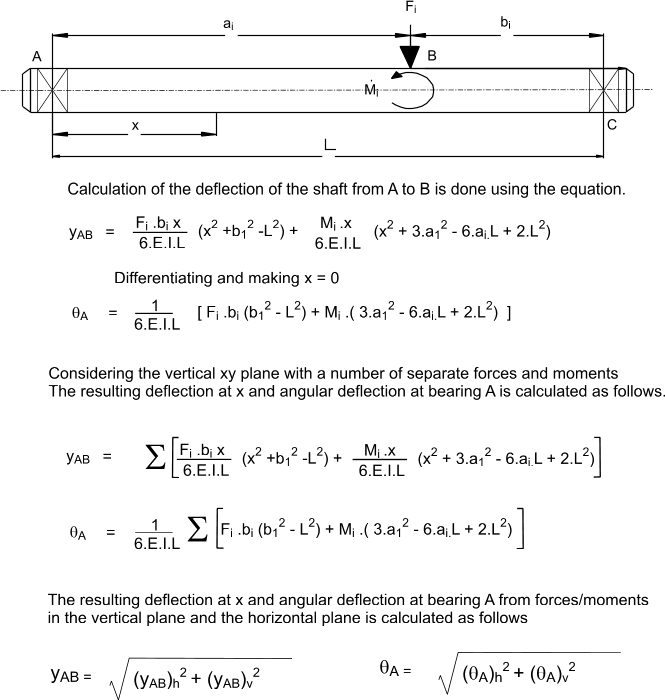Shafts of with many diameters

For more complicated shafts with more than one diameter various methods are available including the Area Moments method ref. Beam Theory and Energy methods (Castigliano's method) .    ref. .Energy Methods    However it is often easier to use numerical integration to obtain shaft deflections which are reasonably accurate.    This basis for this method is the differential equation below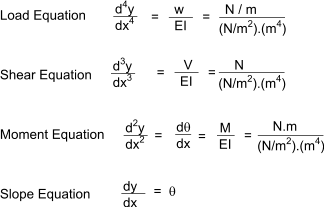.

The simple basis of this method is that the change in slope between two points Δθ = Δx.(M/EI) .... Δx being the distance betwen the points.     The resulting change in deflection is clearly Δθ.Δx.     If this simple rule is applied along the shaft the cummulative slope relative to the initial slope can be evaluated and also the progressive change in deflection.    The smaller the x increments :the more accurate the calculation.     The angles and deflection are all relative to the tangent of the left hand point. If the origin is a bearing then it is clear that the second bearing is at the same radial position as the first bearing.    By simply dividing the calculated overall deflection between the bearings by the centre distance an integration factor (K) results which can be used to convert the relative deflections to absolute by subtracting K.Δ.x from each of the deflection values.

The method is based on one provided in the book "Machine Design: Theory and Practice.. by A.D Deutshman, W.E.Michels, C.E.Wilson, (McMillan Publishing Co Inc)"

A version of the spreadsheet below has be created with the excelcalc.com repository which enables convenient access to the method of calculating deflections of stepped shafts ref ExcelCalcs.com calculation Shaft Deflections

The first step is to evaluate all forces and reactions in the shaft and identify points along the shaft at each force and reaction and change of section. It is also more accurate if long sections of constant diameter with no loads are divided into smaller intervals with associated points.

This method is illustrated using a shaft example below (vertical and horizontal planes ).

 Note:I have completed this example using excel. It takes about 20 minutes to set up the spreadsheet for calculating the deflections in the vertical plane. The table for the deflections in the horizontal plane takes about a minute because the whole table can be cut and pasted and only the forces need to be changed.   Further shaft calculations are then relatively easy because the existing worksheet can easily by used as a template.    I have checked the values against the results from using Mitcalc.com (shafts) and they are reasonably consistent(within 5%). For this example I have not included the weight of the shaft.    The effect of this on the worst case deflection between the bearings is about 0,04mm. (I used Mitcalc.com to check this ).    This clearly shows that for many applications it is not necessary to consider the deflection due to self weight.    For cases where the vertical forces tend the bow the shaft upwards the self weight actually reduces the deflection. - The example below is very much a case where the self weight contributes to the deflection and is still very small compared to the total deflection.     The weights can be reasonably accurately covered by adding the force resulting from the weight of each section to be added at a point midway along the section length.

Shaft Details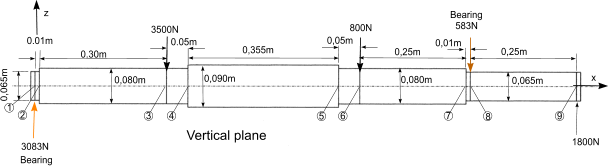Material Steel
E 210.10^9 Pa ( N/m2 )
Dia shaft at points 1-2/7-8; 0,065m (65mm) ..I = 8,762.10-7 m4..IE = 1,84.105.Nm2
Dia shaft at points 2-4/5,7; 0,080m (80mm) ..I = 2,01.10-6 m4..IE = 4,22.105.Nm2
Dia shaft at points 4-5; 0,095m (95mm) ..I = 8,998.10-6 m4..IE = 8,39.105.Nm2
Bearing centre distance = 1,025m (1025mm ). This is used to calculate the total integration constant

The following step by step approach is used to complete this procedure

1) Construct a table with the columns as shown.  Calculate the bearing forces.    Calculate the valuesof EI for each section.      Calculate the bearing centre distance.
2) Divide shaft into intervals with designated points at each force and change of section. If more accuracy is required long then sections of the same diameter can also be divided and allocated point references.
3) List the point number on each alternate row. (space between each point row ).
4) List the forces against the relevant point in column 2. List the distances between the points in column 5. List the EI values in column 6.
5) Calculate the shear force( V)at each point by summing the forces in the preceding rows of column 2 enter (V) in column 3 against the relevant point .
6) Calculate the Bending moment (M = V.x) resulting from the shear force(V) and the distance to the preceding point (x). Add this value to the previous value of M in the point row above and enter this new value of M in column 5 in the current point row.
7) Complicated bit.Calculate M/EI values for each point. Two values are required for each point, one entered in column 7 in the point row and the second in the row below. The first value is M in the point row divided EI in the next row. The second value is M in the next point row divided by the same EI value.
8) Calculate the average of each pair of M/EI values ((Sum of values /2) and enter in column 8 in the row below the point row.
9) Calculate slope (relative to that at point 1) at each point. = slope at previous point + M/EIav. x . Enter this value to column 9 value
10) Calculate the average relative slope between each point (slope x + slope x+1)/2. Enter this in column 10 in the row between the points.
11) Calculate shaft deflection resulting from the average slope by multiplying the slope by the x value in the following row .    This is entered in column 11 in the same row as the average slope
12) Total all the deflection increments between the bearing centres (between rows 1 and 8 in example below). Divide this value by -(bearing centre distance).    This is the integration constant. (K)

Note: The slopes calculated have all been relative to the slope at point 1. They are relative not absolute values.    The resulting calculated deflections are also relative to the tangent of the shaft at point 1 - they are not absolute values.     The total deflection between the bearings is zero and thus the integration constant is the proportional error away from the correct value (error /m) . This is used to correct all of the intermediate values as follows

13) Multiply the total integration constant (K) by the x values between points. Add this relative integration constant value in column 12 in the same row as the deflection increment.

14)The corrected deflection at each point is the product of the relative integration constant and the calculated deflection increment in the preceding row.    Enter this in the point row in column 13.    To this add the corrected deflection of the previous point to make up a running total.

The slopes calculated are also relative to the tangent at point 1. To arrive at the absolute slope /angle at any point simply add the angle correction value. θ c = (- tan-1 ((K) ).

 1 2 3 4 5 6 7 8 9 10 11 12 13 Point on shaft ForceReaction Shear atpreceding point (V) Dist.(x) B.M(M) Flexural rigidity - Average of (1000.M/EI) Slope relative toPoint 1 Average relative. slope Deflection increase Integration constant Deflection F V Lx ∑(V.Lx) EI 1000.M/EI (100M/EI)av θ = ∑Lx(M/EI)av θav δrel=θav.Lx K δ N N m Nm N.m2 mm-1 mm-1 m.rad m.rad mm mm mm 1 3083.41 0 0 0 184011 0.168 0.08 0.001 0 -0.006 2 3083.41 0.01 30.83 0.073 0.001 -0.006 422230 2.264 1.17 0.177 0.053 -0.186 3 -3500 3083.41 0.3 955.85 2.264 0.352 -0.139 422230 2.214 2.24 0.408 0.02 -0.031 4 -416.59 0.05 935.02 1.114 0.464 -0.15 839622 0.937 1.03 0.647 0.23 -0.22 5 -416.59 0.355 787.13 1.864 0.83 -0.14 422230 1.815 1.84 0.876 0.044 -0.031 6 -800 -416.59 0.05 766.3 1.815 0.922 -0.127 422230 1.095 1.46 1.105 0.276 -0.155 7 -1216.59 0.25 462.15 2.512 1.287 -0.006 184011 2.445 2.48 1.299 0.013 -0.006 8 -583.41 -1216.59 0.01 449.98 2.445 1.31 0.001 184011 0 1.22 1.47 0.37 -0.155 9 1800 -1800 0.25 -0.02 1.62 0.216 Total Integration Constant; K = -0,6205mm Angle integration Constant; θ c = -0,62 mrad

To calculate the shaft angle,in the vertical planes, at the bearing centres Points 1 and 8. Simply add θ c to the tabled angles at these points.

Deflections in Horizontal plane

The same method is applicable for obtaining the deflections in the horizontal plane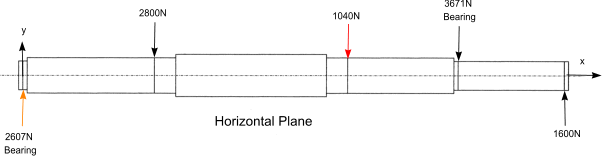1 2 3 4 5 6 7 8 9 10 11 12 13 Point on shaft ForceReaction Shear atpreceding point (V) Dist.(x) B.M(M) Flexural rigidity - Average of (1000.M/EI) Slope relative toPoint 1 Average relative. slope Deflection increase Integration constant Deflection F V Lx ∑(V.Lx) EI 1000.M/EI (100M/EI)av θ = ∑Lx(M/EI)av θav δrel=θav.Lx K δ N N m Nm N.m2 mm-1 mm-1 m.rad m.rad mm mm mm 1 2607,22 0 0 0 184011 0,142 0,071 0,001 0 -0,005 2 2607,22 0,01 26,07 0,062 0,001 -0,005 422230 1,914 0,988 0,149 0,045 -0,161 3 -2800 2607,22 0,3 808,24 1,914 0,297 -0,121 422230 1,891 1,903 0,345 0,017 -0,027 4 -192,78 0,05 798,6 0,951 0,392 -0,131 839622 0,87 0,911 0,554 0,197 -0,191 5 -192,78 0,355 730,16 1,729 0,715 -0,125 422230 1,706 1,718 0,758 0,038 -0,027 6 -1040 -192,78 0,05 720,52 1,706 0,801 -0,114 422230 0,977 1,342 0,969 0,242 -0,134 7 -1232,78 0,25 412,33 2,241 1,137 -0,006 184011 2,174 2,208 1,149 0,011 -0,005 8 -367,22 -1232,78 0,01 400 2,174 1,16 0 184011 0 1,09 1,3 0,33 -0,134 9 1600 -1600 0,25 0 1,43 0,196 Total Integration Constant; K = -0,537 Angle integration Constant; θ c =-0,537 mrad

To calculate the shaft angle,in thehorizontal planes, at the bearing centres Points 1 and 8. Simply add θ c to the tabled angles at these points.

Calculating shaft deflection

The numerical methods above enable calculation of the deflection of the shaft in the horizontal and vertical planes. To obtain the total resultant deflection and the angle of the resultant deflection . The following calculations are completed

δR  =  Sqrt (δH2 + δV 2 )      Angle of resultant from horizontal = tan -1 (δH / δH )

Example..based on shaft above..
The total deflection at point 3 = Sqrt[ (-0.139)2 + (-0.121)2 ] =0,184mm

 Links to Information on designing shaft suppliers and shaft locking methods MITCALC ..A reasonably priced Excel based software package allowing detailed shaft design to European Codes- I recommend this software Machine Design_Stepped shaft deflection ..how to calculate deflection of stepped shaft (the easy way) Method of analysis of stepped shaft ..Detailed Notes using energy methods DANotes ..(U of W australia) Very informative ..Section on Stress Strength and Safety for shafts Chapter 9 Shaft Design ..(Colorado School of Mines) Excellent downloadable chapter of shaft design. Shaft Deflection Calculator .. Convenient calculator for shafts of on dia.

Drive_Index